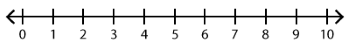##### Notes

Daily Math Practice provides a week's worth of problems ideal for morning work or review. This printable reviews the Kindergarten Operations & Algebraic Thinking and Number & Operations in Base Ten Common Core Mathematics Standards.

##### Print Instructions

NOTE: Only your test content will print.
To preview this test, click on the File menu and select Print Preview.

See our guide on How To Change Browser Print Settings to customize headers and footers before printing.

# Kindergarten-Daily Math Practice-Operations Review (Kindergarten)

Print Test (Only the test content will print)
 DAILY MATH PRACTICE - Operations Review DAY 1 DAY 2 Subtract. Use the number line.9 - 5 = Add. Use the number line.2 + 7 = 5 children were on swings. 2 children got off the swings. How many children were left on the swings? Draw a picture:Answer:children Write the missing numbers.10 += 12+ 6 = 1610 += 18 Circle the pairs that add to 8.5 + 3 7 + 21 + 84 + 4 Write the missing number.3 += 10 Add.2 + 2 = 1 + 4 = Subtract.4 - 3 = 5 - 1 =

 DAILY MATH PRACTICE - Operations Review DAY 3 DAY 4 Subtract. Use the number line.10 - 8 = Add. Use the number line.3 + 4 = There are 6 cups on the shelf. Kenny puts 2 more cups on the shelf. How many cups are on the shelf now? Draw a picture:Answer:cups Write the missing numbers.10 += 17+ 1 = 1110 += 14 Circle the pairs that add to 9.7 + 3 4 + 58 + 23 + 6 Write the missing number.6 += 10 Solve.4 - 2 = 3 + 2 = Solve.2 + 1 = 5 - 3 =You need to be a HelpTeaching.com member to access free printables.